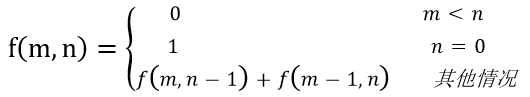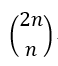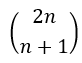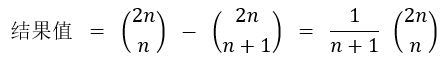组合数学实现关于购票问题的求解以及多种算法实现的性能比较

(在苹果系统下，如果文章中的图片不能正常显示，请升级Safari浏览器到最新版本，或者使用Chrome、Firefox浏览器打开。)

】程序设计中有一类问题是购票问题，在整钱找零钱时怎样的队伍排列可以使得售票处不会出现找不开零钱的局面。本文将采用五种算法分析这道题目，并对各种算法的效率加以比较。从中可以看出组合数学理论在算法优化方面起到的显著作用。

0. 引言

1. 几种算法的实现

1.1. 算法1：搜索策略（暴力破解法）

(实现代码见附件1.py和1.cs)

1.2. 算法2：栈模型

1.3. 算法3：递归算法(DFS)(1)不满足条件的类型，直接终止执行

a<b 或 a>N 或 b>N

(2)当a==b==N时，全部符合条件，结果+1

(3)直接继续分别b+1和a+1递归搜索。

(实现代码见附件3.py和3.cs)

1.4. 算法4：递推算法，动态规划

(实现代码见附件4.py和4.cs)

1.5. 算法5：组合算法——卡特兰数(实现代码见附件5.py和5.cs)

2. 五种算法性能比较

(前3个算法使用C#代码计时，后两个同时使用C#和Python代码计时)

 n 运行结果 T1(n) T2(n) T3(n) T4(n) (C#/Python) T5(n)(C#/Python) 5 42 <0.001 <0.001 <0.001 <0.000001 / 0.036 <0.001 / <0.001 10 16796 0.002 1.154 0.001 <0.001 / 0.041 <0.001 / <0.001 13 742900 0.085 >60.0 0.017 <0.001 / 0.037 <0.001 / <0.001 15 9694845 1.269 >60.0 0.214 <0.001 / 0.038 <0.001 / <0.001 16 35357670 4.913 >60.0 0.788 <0.001 / 0.039 <0.001 / <0.001 17 129644790 19.088 >60.0 2.906 <0.001 / 0.039 <0.001 / <0.001 18 477638700 74.019 >60.0 10.797 <0.001 / 0.038 <0.001 / <0.001 20 6564120420 >60.0 >60.0 >60.0 <0.001 / 0.040 <0.001 / <0.001 30 约3.81*1015 >60.0 >60.0 >60.0 <0.001 / 0.038 <0.001 / <0.001 50 约1.98*1027 >60.0 >60.0 >60.0 <0.001 / 0.041 <0.001 / <0.001 100 约8.965*1056 >60.0 >60.0 >60.0 0.001 / 0.041 <0.001 / <0.001 200 约5.12*10116 >60.0 >60.0 >60.0 溢出 / 0.051 <0.001 / <0.001 300 约4.49*10176 >60.0 >60.0 >60.0 溢出 / 0.069 <0.001 / <0.001 500 约5.39*10296 >60.0 >60.0 >60.0 溢出 / 0.126 <0.001 / <0.001 1000 约2.05*10598 >60.0 >60.0 >60.0 溢出 / 0.427 <0.001 / <0.001

3. 总结

1.cs

/*******************************************
本代码为组合数学大作业题目算法1的实现，C#版本
********************************************/
using System;

namespace zuheshuxue_goupiaowenti
{
public class Program
{
public int N = 0;
public int k = 0;
public int ans = 0;

public static int Main()
{
Program p = new Program();

return 0;
}

public Program()
{
System.Console.WriteLine("请输入数N >>> ");

DateTime beforDT = System.DateTime.Now;

dfs(0);

DateTime afterDT = System.DateTime.Now;
TimeSpan ts = afterDT.Subtract(beforDT);

System.Console.WriteLine("结果是："+ans);
Console.WriteLine("DateTime总共花费{0}ms.", ts.TotalMilliseconds);
}

public void dfs(int tmp)    //回溯
{
if(tmp == 2 * N)    //如果全排完了
{
if(k == 0)    //满足出现了N个100 N个50答案数+1
ans++;
return ;
}
if(k != 0)    //如果还有50的
{
k--;    //就可以让100的来
dfs(tmp + 1);    //深入
k++;    //恢复
}
k++;    //在加一个50的显然不成问题
dfs(tmp + 1);    //搜索
k--;
}
}
}

1.py

#!/usr/bin/env python3
# -*- coding: utf-8 -*-
'''
本代码为组合数学大作业题目算法1的实现，Python版本
'''

global k
k=0
global ans
ans=0

def dfs(N,tmp):    #回溯
global k
global ans

if(int(tmp) == 2 * int(N)):    #如果全排完了
if(k == 0):    #满足出现了N个100 N个50答案数+1
ans+=1
return

if(k != 0):    #如果还有50的
k-=1    #就可以让100的来
dfs(N, tmp + 1)    #深入
k+=1    #恢复

k+=1    #在加一个50的显然不成问题
dfs(N, tmp + 1)    #搜索
k-=1

N = input("请输入数N >>> ")
N = int(N)

dfs(N, 0)
print("总方案数结果：", ans)

3.cs

/*******************************************
本代码为组合数学大作业题目算法3的实现，C#版本
********************************************/
using System;

namespace zuheshuxue_goupiaowenti
{
public class Program
{
public int n = 0;
public int cnt = 0;

public static int Main()
{
Program p = new Program();

return 0;
}

public Program()
{
System.Console.WriteLine("请输入数N >>> ");

DateTime beforDT = System.DateTime.Now;

dfs(0, 0);

DateTime afterDT = System.DateTime.Now;
TimeSpan ts = afterDT.Subtract(beforDT);

System.Console.WriteLine("结果是：" + cnt);
Console.WriteLine("DateTime总共花费{0}ms.", ts.TotalMilliseconds);
}

public void dfs(int a, int b)
{
if(a < b || a > n || b > n)
return;
else if(a == n && b == n)
cnt++;
else
{
dfs(a, b + 1);
dfs(a + 1, b);
}
}
}
}

3.py

#!/usr/bin/env python3
# -*- coding: utf-8 -*-
'''
本代码为组合数学大作业题目算法3的实现，Python版本
'''

global cnt
cnt = 0
global n
n = 0

def dfs(a, b):
global cnt
global n

if(a < b or a > n or b > n):
return
elif(a == n and b == n):
cnt += 1
else:
dfs(a, b + 1)
dfs(a + 1, b)

N = input("请输入数N >>> ")
n = int(N)

dfs(0, 0)
print("总方案数结果：", cnt)

4.cs

/*******************************************
本代码为组合数学大作业题目算法4的实现，C#版本
********************************************/
using System;

namespace zuheshuxue_goupiaowenti
{
public class Program
{

public long[][] dp = new long[];
public int a,b,n;

public static int Main()
{
Program p = new Program();

return 0;
}

public Program()
{
for(int i=0;i<100;i++)
dp[i]=new long;
System.Console.WriteLine("请输入数N >>> ");

DateTime beforDT = System.DateTime.Now;

dp=1;
for(a = 1; a <= n; a++)
{

for(b = 0; b <= a && b <= n; b++)
{
if((b == n || b == a) && a == n)
dp[a][b] = dp[a - 1][b] + dp[a][b - 1] + 1;
else if(b>0)
dp[a][b] = dp[a - 1][b] + dp[a][b - 1];
else
dp[a][b] = dp[a - 1][b];
}
}

DateTime afterDT = System.DateTime.Now;
TimeSpan ts = afterDT.Subtract(beforDT);

System.Console.WriteLine("结果是：" + (dp[n][n]-1));
Console.WriteLine("DateTime总共花费{0}ms.", ts.TotalMilliseconds);
}

}
}

4.py

#!/usr/bin/env python3
# -*- coding: utf-8 -*-
'''
本代码为组合数学大作业题目算法4的实现，Python版本
'''

import datetime

N = input("请输入数N >>> ")
n = int(N)

start = datetime.datetime.now()

dp = [[0 for i in range(1001)] for i in range(1001)]
dp=1

for a in range(1, n + 1) :
b=0
while(b<=a and b<=n):
if((b==n or b==a) and a==n):
dp[a][b]=dp[a-1][b]+dp[a][b-1]+1
elif(b>0):
dp[a][b] = dp[a - 1][b] + dp[a][b - 1];
else:
dp[a][b] = dp[a - 1][b];
b+=1

end = datetime.datetime.now()

print("总方案数结果：", dp[n][n]-1)
print ("总花费时间："+str(end-start))

5.cs

/*******************************************
本代码为组合数学大作业题目算法5的实现，C#版本
********************************************/
using System;

namespace zuheshuxue_goupiaowenti
{
public class Program
{

public int n;

public static int Main()
{
Program p = new Program();

return 0;
}

public Program()
{

System.Console.WriteLine("请输入数N >>> ");

DateTime beforDT = System.DateTime.Now;

long ans = 1;

for(int i = 0; i < n; i++)
ans = ans * (2 * n - i)/(i + 1);

ans/=(n+1);

DateTime afterDT = System.DateTime.Now;
TimeSpan ts = afterDT.Subtract(beforDT);

System.Console.WriteLine("结果是：" + (ans));
Console.WriteLine("DateTime总共花费{0}ms.", ts.TotalMilliseconds);
}

}
}

5.py

#!/usr/bin/env python3
# -*- coding: utf-8 -*-
'''
本代码为组合数学大作业题目算法5的实现，Python版本
'''
import datetime

N = input("请输入数N >>> ")
n = int(N)

start = datetime.datetime.now()

ans = 1

for i in range(0,n):
ans = ans * (2 * n - i)/(i + 1);

ans /= (n + 1)

end = datetime.datetime.now()

print("总方案数结果：", ans)
print("总方案数结果：", int(ans))
print ("总花费时间："+str(end-start))